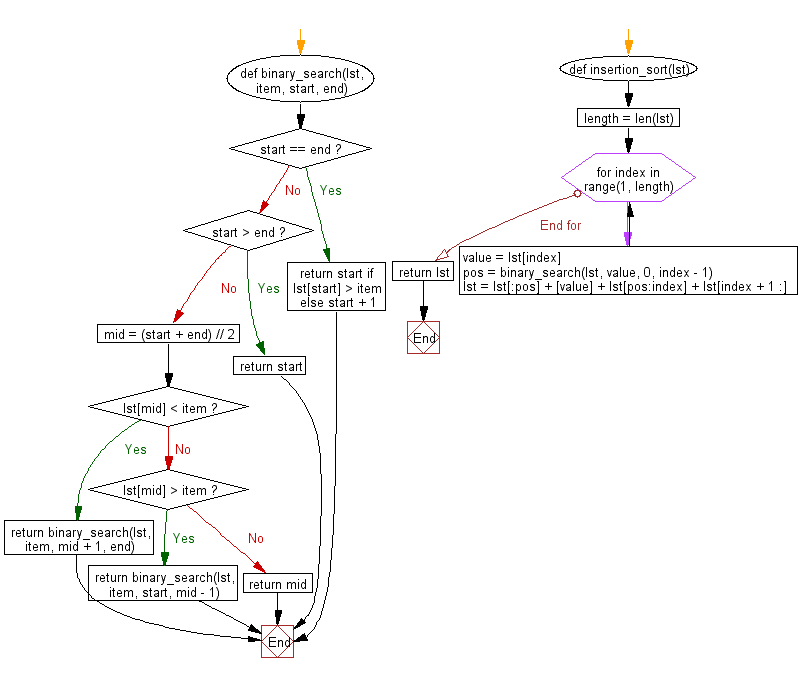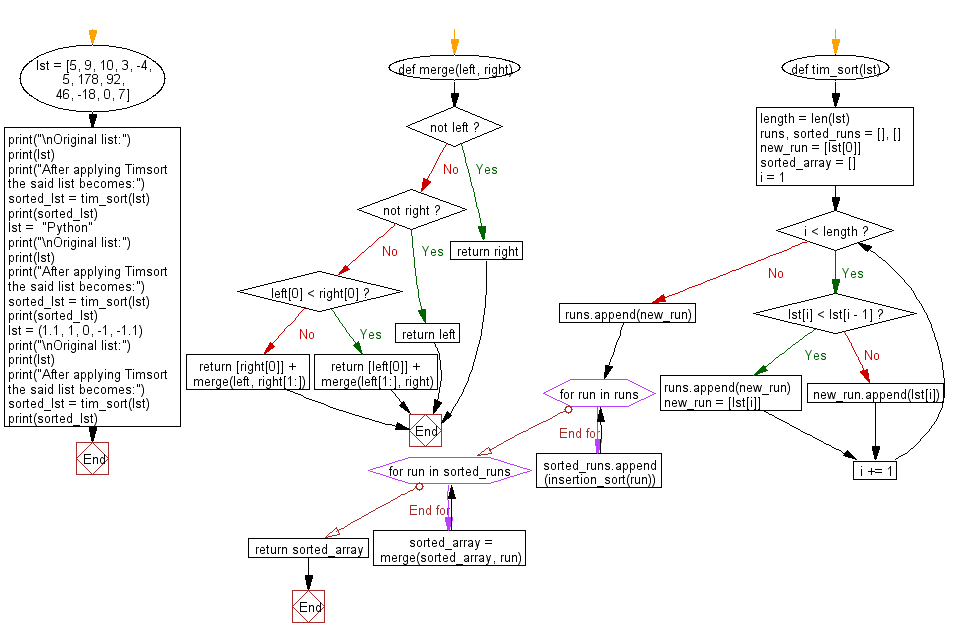﻿ Python: Sort unsorted numbers using Timsort - w3resource# Python: Sort unsorted numbers using Timsort

## Python Search and Sorting : Exercise-25 with Solution

Write a Python program to sort unsorted numbers using Timsort.

From Wikipedia:
Timsort is a hybrid stable sorting algorithm, derived from merge sort and insertion sort, designed to perform well on many kinds of real-world data. It was implemented by Tim Peters in 2002 for use in the Python programming language. The algorithm finds subsequences of the data that are already ordered (runs) and uses them to sort the remainder more efficiently. This is done by merging runs until certain criteria are fulfilled. Timsort has been Python's standard sorting algorithm since version 2.3.

Sample Solution:

Python Code:

``````#Ref:https://bit.ly/3qNYxh9
def binary_search(lst, item, start, end):
if start == end:
return start if lst[start] > item else start + 1
if start > end:
return start

mid = (start + end) // 2
if lst[mid] < item:
return binary_search(lst, item, mid + 1, end)
elif lst[mid] > item:
return binary_search(lst, item, start, mid - 1)
else:
return mid
def insertion_sort(lst):
length = len(lst)
for index in range(1, length):
value = lst[index]
pos = binary_search(lst, value, 0, index - 1)
lst = lst[:pos] + [value] + lst[pos:index] + lst[index + 1 :]
return lst
def merge(left, right):
if not left:
return right

if not right:
return left
if left < right:
return [left] + merge(left[1:], right)
return [right] + merge(left, right[1:])

def tim_sort(lst):
length = len(lst)
runs, sorted_runs = [], []
new_run = [lst]
sorted_array = []
i = 1
while i < length:
if lst[i] < lst[i - 1]:
runs.append(new_run)
new_run = [lst[i]]
else:
new_run.append(lst[i])
i += 1
runs.append(new_run)
for run in runs:
sorted_runs.append(insertion_sort(run))
for run in sorted_runs:
sorted_array = merge(sorted_array, run)
return sorted_array
lst = [5, 9, 10, 3, -4, 5, 178, 92, 46, -18, 0, 7]
print("\nOriginal list:")
print(lst)
print("After applying Timsort the said list becomes:")
sorted_lst = tim_sort(lst)
print(sorted_lst)
lst =  "Python"
print("\nOriginal list:")
print(lst)
print("After applying Timsort the said list becomes:")
sorted_lst = tim_sort(lst)
print(sorted_lst)
lst = (1.1, 1, 0, -1, -1.1)
print("\nOriginal list:")
print(lst)
print("After applying Timsort the said list becomes:")
sorted_lst = tim_sort(lst)
print(sorted_lst)
```
```

Sample Output:

```Original list:
[5, 9, 10, 3, -4, 5, 178, 92, 46, -18, 0, 7]
After applying Timsort the said list becomes:
[-18, -4, 0, 3, 5, 5, 7, 9, 10, 46, 92, 178]

Original list:
Python
After applying Timsort the said list becomes:
['P', 'h', 'n', 'o', 't', 'y']

Original list:
(1.1, 1, 0, -1, -1.1)
After applying Timsort the said list becomes:
[-1.1, -1, 0, 1, 1.1]
```

Flowchart:Python Code Editor:

What is the difficulty level of this exercise?

Test your Programming skills with w3resource's quiz.

﻿

## Python: Tips of the Day

Use Reversed() In for Loops:

```>>> tasks = ['laundry', 'picking up kids', 'gardening', 'cooking']